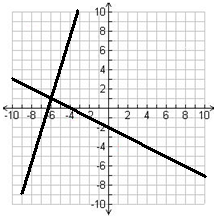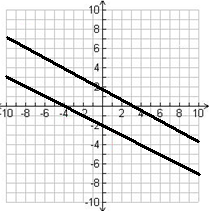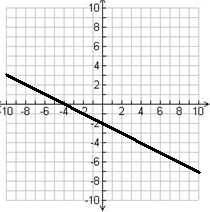# Solving systems of linear equations by graphing

### Solving systems of linear equations by graphing

In order to determine the number of solutions to a system of linear equations, other than by finding the slope of the lines, we can also graph the equations out and look for the intersection of the lines.

#### Lessons

One Solution: Two linear equations intersect at one point. It is a consistent system of independent equation.No Solution:Two linear equations are parallel to each other. It is an inconsistent system.Infinite Solution:• Introduction
a)
How to find the number of solutions to systems of linear equations?

b)
How to solve systems of linear equations by graphing?

• 1.
Graph the following linear equations and find the point of intersection. State the consistency.
a)
2x - y = - 9
x + 3y = 6

b)
2x + 2y = 8
3x - y = 0

c)
4x + 6y = 12
2x + 3y = 9

d)
y - x = 2
4y - 8 = 4x